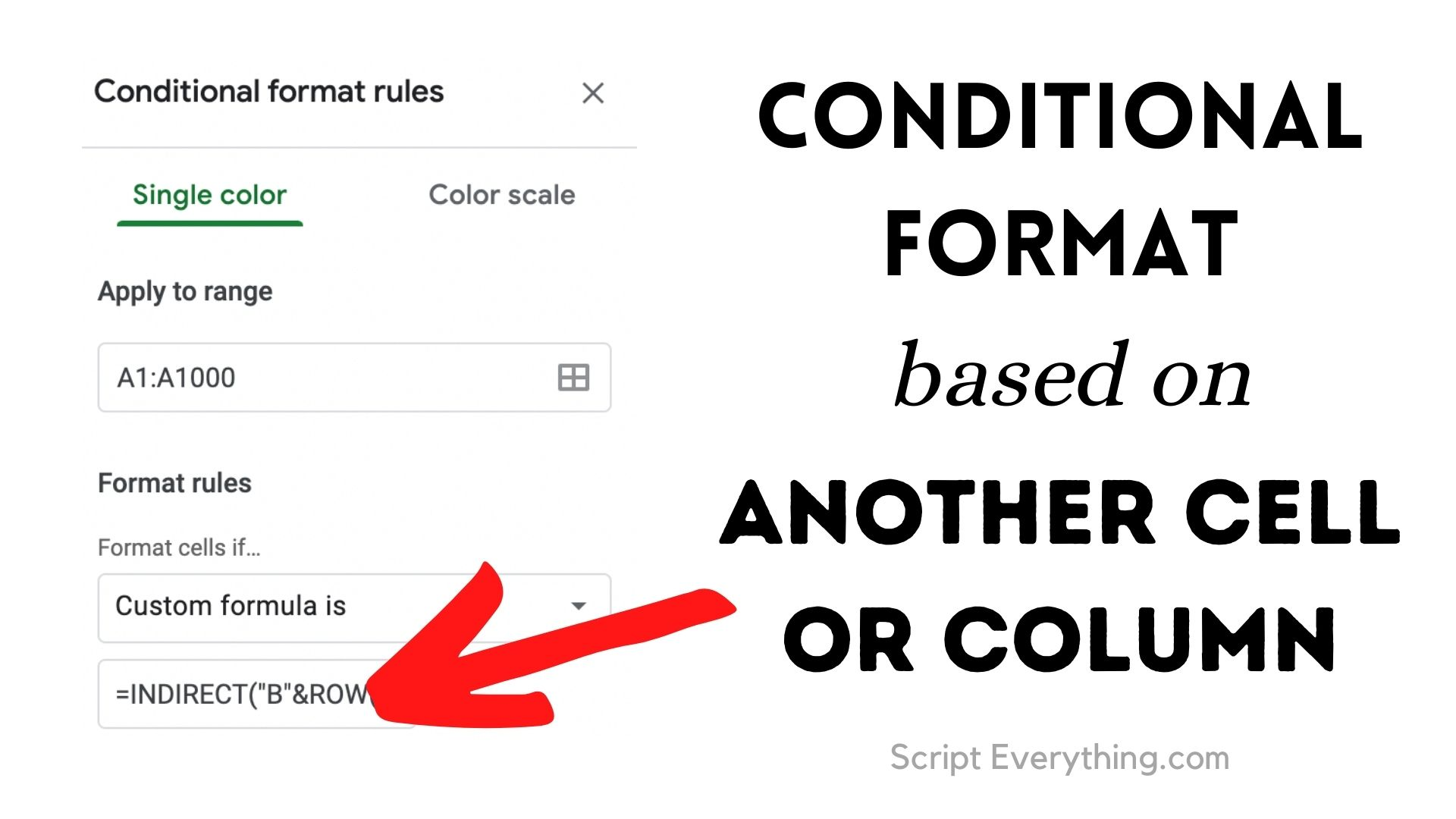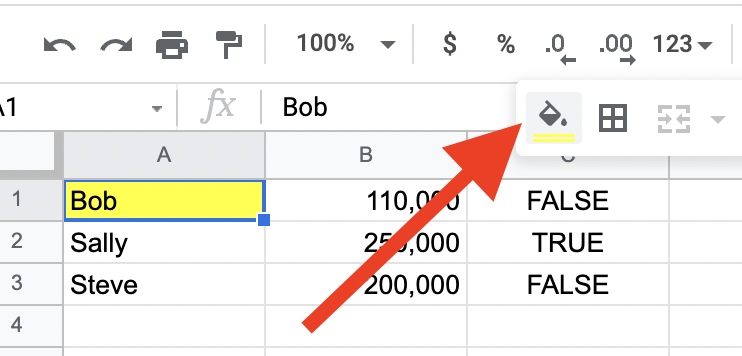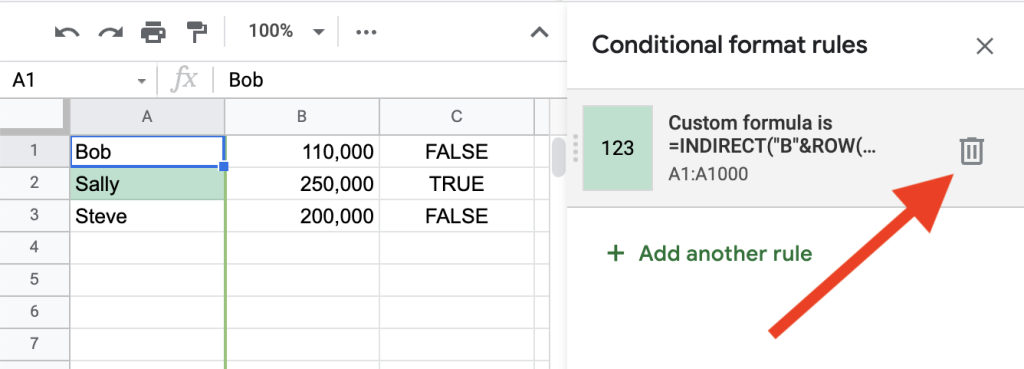# Set Conditional Format Based On Another Cell Value In Google SheetsHow can you create a conditional format in Google Sheets based on the value from another cell or column regardless of whether that cell is on the same sheet or another?

To reference any cell or column in the Custom Formula field in Google Sheets’ conditional formatting section use the ``` INDIRECT() ``` function referencing that cell or adjacent column.

Recently I had a simple requirement where I wanted to highlight a range of cells according to the ``` MONTH() ``` of a cell containing a date value.

For example, I had a cell on a ``` Settings ``` sheet which I gave it the Named Range value of ``` setEndDate ``` . The cells I wanted to highlight had the name of the month and I wanted to highlight these cells that matched the month of the ``` setEndDate ``` field.

I knew my formula would initially look something like this, where ``` A1 ``` was the first cell in the selected range where I wanted the highlighting to start:

``=IF(A1=UPPER(TEXT(setEndDate, "mmm")),TRUE,FALSE)``

Remember when setting a Custom Formula the first cell needs to be inserted into the formula. Google Sheets then automatically replaces this cell reference with the next cell in the range where Conditional Formatting will apply.

This is a very important point as you’ll soon see why.

Here’s what happened when I inserted my formula into the Custom formula is field in the Conditional formatting section, as you can see it didn’t work:

It doesn’t matter whether a Named Range value or the actual range reference (eg. ``` Sheet1!A1 ``` ) is inserted into the formula the result is still the same: ``` Invalid Formula ``` .

## How To Get Around ``` Invalid Formula ``` Error With Cell References

To get around the ``` Invalid Formula ``` error in the Custom Formula field in the Conditional Formatting section simply replace the cell reference (whether an actual reference or Named Range value) by wrapping it into the ``` INDIRECT() ``` formula.

The ``` INDIRECT() ``` function translates the value inserted into the parameters of the function into a value that is used by the remaining formula, so my initial function changed to this:

``=IF(A1=UPPER(TEXT(INDIRECT("setEndDate"), "mmm")),TRUE,FALSE)``

And this enabled the Custom Function to work.

## Conditional Formatting And ``` IF ``` Statement

The purpose of a Custom Formula used in a conditional format is to structure the formula so that it is a boolean operation. While you can use an ``` IF() ``` statement as above it needs to return boolean values so Google Sheets knows when to apply the format.

Therefore, if the ``` IF() ``` function is already returning boolean values and in the correct order (i.e. ``` TRUE ``` is the first value returned after the statement, and ``` FALSE ``` is the last value returned) then the ``` IF() ``` statement is redundant and can be removed.

My custom formula would now look like this:

``=A1=UPPER(TEXT(INDIRECT("setEndDate"), "mmm"))``

If your ``` IF() ``` function has the boolean values swapped – i.e. ``` IF(statement,FALSE,TRUE) ``` then you still can remove the ``` IF() ``` statement and instead wrap your ``` statement ``` section with the function ``` NOT() ``` .

For example, if I wanted to highlight cells that were not empty using a custom formula I could write the following:

``=NOT(A1="")``

This conditional format works on the range where it is applied (in ``` A1:A138 ``` ) and will highlight the cell if the cell is not empty.

The function ``` NOT() ``` simply reverses the boolean result inside. The original function inside states ``` A1 ``` is empty, therefore, should a cell be empty the ``` NOT() ``` function returns ``` FALSE ``` whereas if a cell is not empty, being ``` FALSE ``` inside, the ``` NOT() ``` function returns ``` TRUE ``` .

## Why Use ``` INDIRECT ``` For Custom Formula?

The reason why the ``` INDIRECT() ``` function is needed in the Custom Formula is because of the replacement by Google Sheets of cell references when it applies the logic to other ranges in the Conditional Formatting range.

With my formula above you can see that there is one cell reference inserted into the formula, in my case, the cell ``` A1 ``` :

``=A1=UPPER(TEXT(INDIRECT("setEndDate"), "mmm"))``

And the Apply to range field (as shown in the picture above) is set to ``` A1:A138 ``` .

Therefore, when Google Sheets applies my Custom Formula to the next cell in my ``` A1:A138 ``` range it finds the only cell reference in the formula and replaces it with the next cell, therefore, ``` A2 ``` would look like this for my custom formula:

``=A2=UPPER(TEXT(INDIRECT("setEndDate"), "mmm"))``

Hopefully, you can see why inserting more than one cell reference into a custom formula does not work, and the only way to get a formula to work where other cells are referenced is to use the ``` INDIRECT() ``` function.

## Conditional Format Based On Another Column

What if there were data in an adjacent column as you tracked down the column you wanted to perform the conditional formatting? Can the Custom Formula use adjacent or same length columns to perform a comparison?

Suppose you have the following set of data in your spreadsheet which lists the total amount of widgets sold in a month per sales staff member:

If you wanted to highlight the name of the staff member who had sold more than 200,000 you could use a Custom Formula on column A. Upon selecting all the cells you want the Conditional Formatting to apply insert the following formula:

``=INDIRECT("B"&ROW(A1))>200000``

This formula uses the handy ``` INDIRECT() ``` formula and starts by using the column name ``` "B" ``` where the information is stored to compare and then joins it to the corresponding cell used by Google Sheets in the range for the conditional formatting to be applied. As the range is ``` A1:A1000 ``` Google Sheets will replace this cell with each cell in the range.

Here’s how this looks:

You could further change the formula by creating another cell and setting the Named Range of that cell to ``` salesTarget ``` then your Custom Formula in the Conditional Formatting could reference an additional cell:

``=INDIRECT("B"&ROW(A1))>INDIRECT("salesTarget")``

The important thing to remember when inserting a Custom Formula into the conditional formatting section is that there can only be one cell reference and this must be within the range to whom the formatting is applied.

## Google Sheets Relative Referencing With Conditional Formatting

The ``` INDIRECT() ``` formula can also take relative references. Instead of using ``` "B"&ROW(A1) ``` you can also use the distance in rows or columns a range is from the active cell. This technique is known as relative referencing .

To use relative referencing in the ``` INDIRECT() ``` formula simply insert the relative reference using the distance in rows and columns away from the active cell.

For example, if the active cell were ``` A1 ``` and you were to use the ``` INDIRECT ``` formula with the relative reference of ``` R1C1 ``` in the active cell, this relative reference would increase the row by 1, and increase the column by 1. Therefore, this relative reference would copy the contents of cell ``` B2 ``` .

To use the same row as the active cell don’t insert any numbers after the ``` R ``` tag in the relative reference.

Therefore, continuing with the example above, instead of using ``` INDIRECT("B"&ROW(A1)) ``` in the custom conditional formula, you could substitute this with the relative reference:

``=INDIRECT("RC1",FALSE)>INDIRECT("salesTarget")``

Notice with the first instance of the ``` INDIRECT ``` formula in the above condition that the parameters used in this formula have changed to ``` RC1 ``` and a second parameter ``` FALSE ``` has been added to assert that the indirect formula reference is a relative reference that does NOT use A1 notation (by default this parameter is set to ``` TRUE ``` ).

Depending on your use case and which you prefer to use, relative referencing can just as easily be used and swapped out for ``` A1 ``` type referencing when using the ``` INDIRECT() ``` formula.

## Conditional Format Custom Formulas: Common Errors

If you find your formula isn’t highlighting the right cells after you’ve entered your new Custom Formula there are a couple of things to check:

1. Is the formula correct?

Test your formula by inserting it somewhere on your sheet and seeing if it does work. In the case of the example above with the Sales staff I copied the formula into an adjacent column and checked to see if it would work, and it’s very easy to do, as shown below:

Copy the same formula used in the Custom Formula into the top most cell and then copy this cell down checking that the cell reference ( ``` A1 ``` ) changes too.

2. Is the cell reference in the Custom Formula “Apply to range” range?

Check the Apply to range field and verify the cell contained within your Custom formula will actually be found in that range.

For example, if the formula contained references to ``` B1 ``` instead of ``` A1 ``` (which is an easy mistake to make when you’re thinking about other columns!) then this will not work as anticipated.

3. Is the cell reference in the Custom Formula starting in upper-left-most range?

Check your cell reference in the Custom Formula matches the first cell range in the Apply To Range field. You can get strange behaviours if you use other cell references, so check the cell reference is the first cell in the range.

4. The background colour doesn’t match the conditional formatting set?

If you have a cell in your conditional format range that has a background colour not matching the rules of the format then this could be due to the cell’s background being set manually .

Simply look for the Fill Color icon and see if the background colour has a colour matching what you are seeing. If so, remove the background colour so that it doesn’t conflict with any conditional format rules you have set for the same range.If background colour matches a condition’s colour, but cell doesn’t match the condition, check if Fill Color has been set manually for the cell

## How To Remove Conditional Formatting

To remove a conditional format click first on a cell where you know formatting is applied, or, if in doubt, select all cells in the active sheet. Then click on the Format menu item followed by the Conditional formatting sub-menu item.

This will bring up a sidebar of the conditional formats available for either the selected cell or all cells you have selected.

To remove the desired condition simply hover your mouse over the row containing the formatting rules and you will see a rubbish bin icon appear and a tooltip will pop up stating Remove rule . To remove the rule simply click this button and the rules for the format will be deleted.From Format > Conditional Formatting simply hover over the rule you wish to delete and click on the trash can icon

If you can’t find a conditional format rule try selecting all cells in the active sheet (click that grey box which doesn’t have any letters or numbers in it – it’s found to the left of label ``` A ``` for column A, and above label ``` 1 ``` for row 1). Here’s what this looks like when you do:If you cannot find a conditional format rule on a sheet that you know would have something…

## Summary

To enable references in the Custom Function field in the Conditional Formatting section of Google Sheets, insert either the reference or the Named Range into the ``` INDIRECT() ``` function.

The reason why the ``` INDIRECT() ``` function is needed is that only one cell reference can be used in the formula for Google Sheets to replace and apply the formula to other cells in the conditional formatting range.

Author of scripteverything.com, Ryan has been dabbling in code since the late '90s when he cut his teeth by exploring VBA in Excel when trying to do something more. Having his eyes opened with the potential of automating repetitive tasks, he expanded to Python and then moved over to scripting languages such as HTML, CSS, Javascript and PHP. When he is not behind a screen, Ryan enjoys a good bush walk with the family during the cooler months, and going with them to the beach during the warmer months.